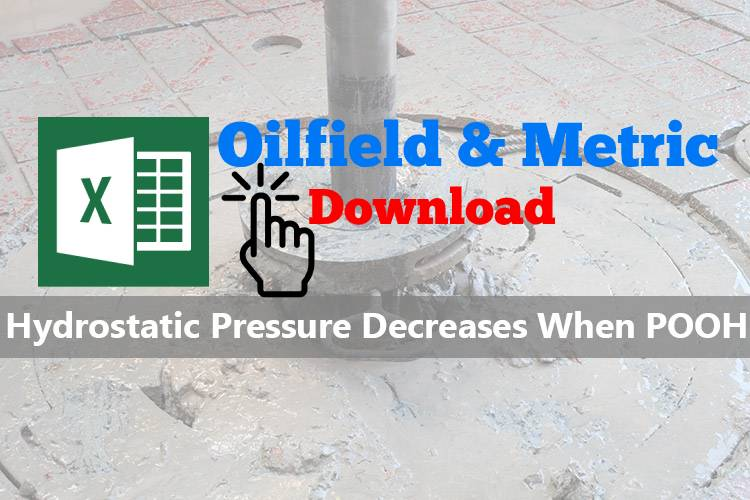# Hydrostatic Pressure (HP) Decreases When POOH

When pulling out of hole, volume of steel will be out of hole and mud volume will replace the steel volume.  If we don’t fill hole, hydrostatic pressure will decrease.  This topic shows you how to calculate hydrostatic pressure loss while pulling out of hole without filling the wellbore.  Moreover, there is the Excel sheet for calculating pressure decrease due to pulling out of hole.

## Oilfield Unit

Step 1: Determine Total Volume of Steel Out of Hole

Total Volume of Steel Out of Hole = Length  of pipe pulled out × Pipe Displacement

Where,

Total Volume of Steel Out of Hole in bbl

Length  of pipe pulled out in ft

Pipe Displacement in bbl/ft

Step 2: Determine Hydrostatic Pressure Decrease

Hydrostatic Pressure Decrease = (Total Volume of Steel Out of Hole × 0.052 × mud weight) ÷ (casing capacity – pipe displacement)

Where,

Hydrostatic Pressure Decrease in psi

Total Volume of Steel Out of Hole in bbl

mud weight in ppg

casing capacity in bbl/ft

pipe displacement in bbl/ft

Example: Determine the hydrostatic pressure decrease when pulling pipe out of the hole:

Number of stands pulled = 10
Pipe displacement = 0.0055 bbl/ft
Average length per stand = 91 ft
Casing capacity = 0.0873 bbl/ft
Mud weight = 12.0 ppg

Step 1: Determine of pipe displacement in Barrels = 10 stands × 91 ft/std × 0.0055 bbl/ft displaced

Total Volume of Steel Out of Hole = 5.01 bbl

Step 2: Determine HP, psi decrease = 5.01 barrels × 0.052 × 12.0 ppg ÷ (0.0873 bbl/ft – 0.0055 bbl/ft)

Hydrostatic pressure decrease = 38.2 psi

## Metric Unit

Step 1: Determine Total Volume of Steel Out of Hole

Total Volume of Steel Out of Hole = Length  of pipe pulled out × Pipe Displacement

Where,

Total Volume of Steel Out of Hole in m3

Length  of pipe pulled out in m

Pipe Displacement in m3 /m

Step 2: Determine Hydrostatic Pressure Decrease

Hydrostatic Pressure Decrease = (Total Volume of Steel Out of Hole × 0.00981 × mud weight) ÷ (casing capacity – pipe displacement)

Where,

Hydrostatic Pressure Decrease in KPa

Total Volume of Steel Out of Hole in m3

mud weight in kg/m3

casing capacity in m3 /m

pipe displacement in m3 /m

Example: Determine the hydrostatic pressure decrease when pulling pipe out of the hole:

Number of stands pulled = 10
Pipe displacement = 0.00287 m3 /m
Average length per stand = 30 m
Casing capacity = 0.04554 m3 /m
Mud weight = 1440 kg/m3

Step 1: Determine of pipe displacement in m3 = 10 stands × 30 m/std × 0.00287 m3 /m pipe displacement

Total Volume of Steel Out of Hole 0.86  m3

Step 2: Determine HP, psi decrease = 0.86  m3 × 0.00981 × 1440 kg/m3 ÷ (0.04554 m3 /m- 0.00287 m3 /m)

Hydrostatic pressure decrease = 285 KPa

Please find the Excel sheet for calculating pressure decrease due to pulling out of hole.Ref books:

Lapeyrouse, N.J., 2002. Formulas and calculations for drilling, production and workover, Boston: Gulf Professional publishing.

Bourgoyne, A.J.T., Chenevert , M.E. & Millheim, K.K., 1986. SPE Textbook Series, Volume 2: Applied Drilling Engineering, Society of Petroleum Engineers.

Mitchell, R.F., Miska, S. & Aadny, B.S., 2011. Fundamentals of drilling engineering, Richardson, TX: Society of Petroleum Engineers.

Share the joyWorking in the oil field and loving to share knowledge.

### 4 Responses to Hydrostatic Pressure (HP) Decreases When POOH

1.Kelly Brown says:

The article is usefull for me. I’ll be coming back to your blog.

2.vignesh says:

Hello,
For a 2 3/8″ N80 tubing string what would be the casing size be. I am asking this as I am solving a problem on the hydrostatic pressure loss of pulling 20, 90′ 2 3/8″ N80 tubings from a depth of 10000′ @ fluid density of 10ppg & formation pressure of 5000psi. The casing size is not provided in this case. I tried finding out the suitable casing size for this tubing but was not successful. If possible please provide some inputs/guidance. thanks for your time.
regards
Vignesh

3.Muhammad Usman says: